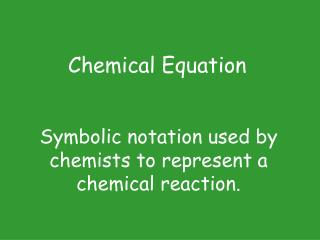DownloadDownload PresentationSymbolic notation used by chemists to represent a chemical reaction.

# Symbolic notation used by chemists to represent a chemical reaction.

Download Presentation## Symbolic notation used by chemists to represent a chemical reaction.

- - - - - - - - - - - - - - - - - - - - - - - - - - - E N D - - - - - - - - - - - - - - - - - - - - - - - - - - -
##### Presentation Transcript

1. Symbolic notation used by chemists to represent a chemical reaction. Chemical Equation

2. In a chemical equation, everything to the left of the arrow Reactant – starting materials

3. In a chemical equation, everything to the right of the arrow Product – ending materials

4. Coefficient Number in front of a formula. Tells how many formula units are present.

5. Balanced chemical equation Demonstrates conservation of mass. Same # of each element on both sides.

6. Chemical equations demonstrate conservation of mass, charge (& energy)

7. Reaction with 1 product Synthesis

8. Reaction with 1 reactant Decomposition

9. Reaction of 1 element with 1 compound to produce a new element & a new compound Single Replacement

10. Reaction of 2 compounds to produce 2 new compounds. Double Replacement

11. AB  A + B Decomposition

12. A + B  AB Synthesis

13. AX + B  A + BX Single Replacement

14. AX + BY  AY + BX Double Replacement

15. A + O2 Products Combustion

16. To predict if a single replacement reaction will occur, You must compare the reactivity of the free element with the corresponding element in the compound. Use Table J. If the stand-alone element is above the corresponding element in the compound, the reaction will take place.

17. Na + MgCl2 ? 2Na + MgCl2 2NaCl + Mg Compare Na & Mg (both metals). Na is above Mg in Table J so the rxn occurs.

18. F2 + MgCl2 ? F2 + MgCl2 MgF2 + Cl2 Compare F2 & Cl2 (both nonmetals). F2 is above Cl2 in Table J so the rxn occurs.

19. Do the following reactions occur? No • Br2 + HF: Compare Br2 & F2 • Mg + ZnCl2: Compare Mg & Zn • Na + HCl: Compare Na & H2 • Ag + LiBr: Compare Ag & Li Yes Yes No

20. To predict if a double replacement reaction will occur, You must determine the possible products & determine if the reaction goes to completion. The reaction goes to completion if a gas, a solid, or a small covalent molecule (H2O) is formed. Use Table F to determine if a precipitate (solid) is formed.

21. To predict if a double replacement reaction will produce a precipitate … Write formulas for the possible products. Use Table F to determine if a precipitate (solid) is formed. Scan through table F looking for information about the solubility of the products.

22. Ba(NO3)2(aq) + Fe2(SO4)3(aq)  ? Write formulas for the possible products: Fe(NO3)3 + BaSO4 Scan through table F looking for information about the solubility of the products: All nitrates are soluble so Fe(NO3)3 is aqueous. Most sulfates are soluble except when combined with Ag+, Ca2+, Sr2+, Ba2+, & Pb2+ so BaSO4 is solid.

23. Stoichiometry Problems Use the relationship in the balanced equation to predict amounts consumed or produced. Use the equation coefficients to set up a ratio.

24. Stoichiometry Problems May involve mole-mole relationships. For reactions involving all gases, coefficients in equation also give information about volume-volume ratios.

25. 2Na + 2H2O  2NaOH + H2How many moles of hydrogen are produced when 4 moles of sodium reacts completely? X 4 2 Moles of H2! 4/2 = X/1 so X = 2 moles

26. CH4(g) + 2 O2(g)  CO2(g) + 2 H2O(g)How many liters of CO2 are produced when 3 liters of CH4 reacts completely? 3 X 3 Liters of CO2! 3/1 = X/1 so X = 3